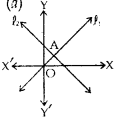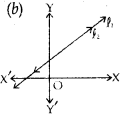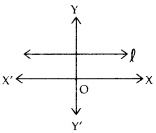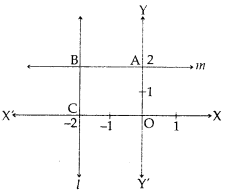# MCQ Questions for Class 10 Maths Chapter 3 Pair of Linear Equations in Two Variables with Answers

Students can access the NCERT MCQ Questions for Class 10 Maths Chapter 3 Pair of Linear Equations in Two Variables with Answers Pdf free download aids in your exam preparation and you can get a good hold of the chapter. Use MCQ Questions for Class 10 Maths with Answers during preparation and score maximum marks in the exam. Students can download the Pair of Linear Equations in Two Variables Class 10 MCQs Questions with Answers from here and test their problem-solving skills. Clear all the fundamentals and prepare thoroughly for the exam taking help from Class 10 Maths Chapter 3 Pair of Linear Equations in Two Variables Objective Questions.

## Pair of Linear Equations in Two Variables Class 10 MCQs Questions with Answers

Students are advised to solve the Pair of Linear Equations in Two Variables Multiple Choice Questions of Class 10 Maths to know different concepts. Practicing the MCQ Questions on Pair of Linear Equations in Two Variables Class 10 with answers will boost your confidence thereby helping you score well in the exam.

Explore numerous MCQ Questions of Pair of Linear Equations in Two Variables Class 10 with answers provided with detailed solutions by looking below.

Question 1.
Find the dimensions of a garden, whose length is 4 m more than its width and half the perimeter is 36 m.
(a) 20 m × 16 m
(b) 14 m × 10 m
(c) 26 m × 22 m
(d) 12 m × 8 m

Answer: (a) 20 m × 16 m
l = b + 4
l + b = 36
l = 20 and, b = 6

Question 2.
The sides of a triangle are AB, BC and AC whose equations are respectively y-2 = 0; y = 3x – 7 and 2y + x = 0, The coordinates of points A and B are :
(a) A(3,2), B(-4,2)
(b) A(-4,2), B(3,2)
(c) A(3,2), B(2,-l)
(d) A(-4,2), B(2, -1)

Equation of line AB : y – 2 = 0 Equation of line BC: y = 3 x -7 Equation of line AC: 2y + x = 0 Coordinates of point B can be obtained by solving AB and BC => (3,2).
Coordinate of point A are (- 4, 2)
A (- 4,2) and B(3,2)

Question 3.
Given is the system of Inconsistent equations, 2x + 7y = 11 and 5x + ky – 25 = 0, find k.
(a) – $$\frac{35}{2}$$
(b) 4
(c) $$\frac{35}{2}$$
(d) – 4

Answer: (c) $$\frac{35}{2}$$
$$\frac{a1}{a2}$$ = $$\frac{b1}{b2}$$ ≠ $$\frac{c1}{c2}$$
$$\frac{2}{5}$$ = $$\frac{7}{k}$$
2k = 35
k = $$\frac{35}{2}$$

Question 4.
10 students of class X took part in a Mathematics quiz. If the number of girls is 4 more than the number of boys, find the number of boys who took part in the quiz.
(a) 3
(b) 7
(c) 2
(d) 8

No. of boys = x
and No. of girls = y
x + y =10
y = x + 4
x = 3
y = 7
=> Number of boys = 3

Question 5.
5 pencils and 7 pens together cost Rs. 50 whereas 7 pencils and 5 pens cost Rs. 46. Find the cost of 2 pencils and 3 pens.
(a) Rs. 42
(b) Rs. 39
(c) Rs. 21
(d) Rs. 16

Let, cost of 1 pencil = x and cost of 1 pen = y – 2
Cost of 2 pencil and 3 pens = 6 +15 = Rs. 21
=> Correct option is (c)

Question 6.
A linear equation in two variables has:
(a) 1 solution
(b) 2 solutions
(c) no solution
(d) infinitely many

A linear equation in two variables has infinite number of solutions.
=> Correct option is (d)

Question 7.
A pair of linear equations is not consistent if:
(a) if has one solution
(b) it has many solutions
(c) graph intersect or coincide
(d) graph is parallel

37. Correct option is (d)

Question 8.
In a AABC, ∠A = x°, ∠B = (3a – 2)° and ∠C = y°. Also, ∠C – ∠B = 9°. Find the value of ∠B.
(a) 73°
(b) 82°
(c) 25°
(d) 49°

∠A + ∠B + ∠C = 180°
=> x + (3x – 2) + y = 180
4x + y = 182 …(1)
y – 3x + 2 = 9 …(2)
Solving (1) & (2), y = 82, x = 25° => ∠B = 73°

Question 9.
The perimeter of a rectangle is 44 cm. Its length exceeds twice its breadth by 4 cm. Find the area of the rectangle.
(a) 46 cm²
(b) 49 cm²
(c) 96 cm²
(d) 69 cm²

Let, Length = x cm
=> 2(x + y) = 44
=> x + y =221, y = 6 cm ,x = 16 cm
x = 2y+4
=> Area = 16 x 6 = 96 cm²

Question 10.
For what value of k, will the equations, x + 2y + (11 – k) = 0 and 2x + ky + (10 + k) = 0 represent the coincident lines :
(a) k = 12
(b) k = 4
(c) k = 36
(d) k = 2

$$\frac{a1}{a2}$$ = $$\frac{b1}{b2}$$ = $$\frac{c1}{c2}$$
$$\frac{1}{2}$$ = $$\frac{2}{k}$$ = $$\frac{11 – k}{10 + k}$$
k = 4

Question 11.
An equation ax + by + c = 0 is a linear equation in 2 variables, where a, b, c are :
(a) natural numbers
(b) whole numbers
(c) integers
(d) real numbers

Question 12.
Read the following graphs carefully, and match the following:Lines do not intersect Unique solutions Consistent with many solutionsLines intersect at 1 point Infinite solutions ConsistentLines are coincident No solutions Parallel linesLines intersect at 1 point Unique solutions ConsistentLines are coincident Infinite solutions Consistent with many solutionsLines do not intersect No solutions Parallel lines

Question 13.
The pair of equations y = 9 and y = – 7 has:
(a) one solution
(b) two solutions
(c) infinitely many
(d) no solution solutions

y = 9 and y = – 7 are lines parallel to X-axis, thus these are parallel lines and hence no solution

Question 14.
The system of equations 3x – 5y = 20 and 6x – 10y = 40 has :
(a) one solution
(b) exactly 2 solutions
(c) infinitely many solutions
(d) no solutions solutions

$$\frac{a1}{a2}$$ = $$\frac{b1}{b2}$$ = $$\frac{c1}{c2}$$ = $$\frac{x}{y}$$

Question 15.
For what value of a, the pair of equations, 3x + 2y – 4= 0 and ax – y – 3 = 0 has a unique solutions :
(a) All real numbers except – $$\frac{3}{2}$$
(b) a=- $$\frac{3}{2}$$
(c)a= $$\frac{3}{2}$$
(d)a= $$\frac{2}{3}$$

Answer: (a) All real numbers except – $$\frac{3}{2}$$
For unique solutions , $$\frac{a1}{a2}$$ ≠ $$\frac{b1}{b2}$$
a ≠ – $$\frac{3}{2}$$
a can all possible values except – $$\frac{3}{2}$$

Question 16.
The sum of a two digit number is 8. The number obtained by reversing the digits exceeds the number by 18. Then the given number is :
(a) 53
(b) 35
(c) 26
(d) 62

Let, the digit at units’ place = x and digit at ten’s place = y => x + y = 8 and lOx + y = 18 + (10y + x)
No. = 35.

Question 17.
In a triangle, the sum of two angles is equal to the third angle. If the difference between two angles is 30°, find the angles.
(a) 15°, 45°, 75°
(b) 20°, 50°, 80°
(c) 30°, 60°, 90°
(d) 45°, 45°, 90°

(x) + (y) + (x + y) 180°
x + y = 90°
x – y = 30°
=> x = 60°, y = 30°
=> Angles are 30°, 60° and 90°

Read the graph carefully and answer the questions given (Q.No. 18 to Q. No. 22):

Question 18.
(a) (0,1)
(b) (4,0)
(c) (2,3)
(d) (0,6)

Correct option is (b).

Question 19.
The line x – y + 1 = 0 meets Y-axis at:
(a) (0,1)
(b) (4,0)
(c) (2,3)
(d) (0,6)

Correct option is (a).

Question 20.
The solutions of the system of equations, x – y + 1 = 0, 3x + 2y -12 = 0 is :
(a) (0,1)
(b) (4,0)
(c) (2,3)
(d) (0,6)

Since the two lines meet at (2, 3)
Correct option is (c).

Question 21.
The area of triangle formed by x-y +1 = 0, 3x + 2y -12 = 0 and X-axis is :
(a) $$\frac{15}{2}$$ sq. units
(b) 9 sq. units
(c) 5 sq. units
(d) 6 sq. units

Answer: (a) $$\frac{15}{2}$$ sq. units
Area of ∆ formed by x – y +1 = 0,3x + 2y – 12 = 0 and X-axis is, (∆ABC)
$$\frac{1}{2}$$ × 5 × 3 = $$\frac{15}{2}$$sq. units

Question 22.
The area of triangl e formed by x – y +1 = 0, 3x + 2y -12 = 0 and x = 0 is :
(a) $$\frac{15}{2}$$ sq. units
(b) 9 sq. units
(c) 5 sq. units
(d) 6 sq. units

Area required = Area (∆ECD)
= $$\frac{15}{2}$$ × 5 × 2 = 5 sq. units

Question 23.
Find the value of (x + y) if 148x + 231y=527 and 231x + 148y = 610.
(a) 3
(b) 480
(c) 1
(d) 481

379x + 379y = 1137
x + y = 3

Question 24.
The line x = 4 :
(a) passes through origin
(b) parallel to X-axis
(c) parallel to Y-axis
(d) does not meet Y-axis at all

x = 4 is a line parallel to Y-axisQuestion 25.
If x and y satisfy the given two equations, 4x + $$\frac{6}{y}$$ = 15, 6x – $$\frac{8}{y}$$ = 14; find p is y = px – 2.
(a) 1
(b) 2
(c) 3
(d) $$\frac{4}{3}$$

Answer: (d) $$\frac{4}{3}$$
4x + $$\frac{6}{y}$$ = 15
6x – $$\frac{8}{y}$$= 14
=> x = 3
=> y = px – 2
y = 2
=> 2 = 3p – 2
=> Correct option is (d).

Question 26.
Find the value which k cannot take, for the given system to have a unique solutions, x + 2y = 3, ky + 5x + 7 = 0.
(a) 10
(b) $$\frac{- 14}{3}$$
(c) any real
(d) any real number
number except 10.

$$\frac{a1}{a2}$$ = $$\frac{1}{5}$$, $$\frac{b1}{b2}$$ = $$\frac{2}{k}$$, $$\frac{c1}{c2}$$ = – $$\frac{3}{7}$$
$$\frac{a1}{a2}$$ ≠ $$\frac{b1}{b2}$$
k ≠ 10

Question 27.
Find p and q so that given system has infinite number of solutions, 4x + 6y -14 = 0.
(p + q)x + (2 p – q)y – 3 (p + q +1) = 0
(a)p = 1, q = 1
(b)p = 1,q = – 1
(c) p = 1, q = 5
(d) p = 5, q = 1

Answer: (d) p = 5, q = 1
System will have infinite solutions, if
$$\frac{b1}{b2}$$ = $$\frac{b1}{b2}$$ = $$\frac{b1}{b2}$$
p – 5q = 0 and p + q = 6
p = 5
q = 1
=> Correct option is (d).

Question 28.
Find x and y in the given rectangle :(a) x = 1, y = 4
(b) x = 4, y = 1
(c) x = 2, y = 2
(d) x = 3, y = 1

Answer: (a) x = 1, y = 4
3x + y = 7 => 3x + y = 7
x + 3y = 13 => 3x + 9y = 39
x = 1; y = 4

Read the figure carefully and answer fhe following questions (Q. No. 29 to Q. No. 33):Question 29.
Equation of line l is,
(a) x = – 2
(b) x = 2
(c)y = 2
(d) y = – 2

Answer: (a) x = – 2
Line l is parallel to Y-axis at a distance of 2 units in the negative direction
=> Equation of line / is x = – 2
=> Correct option is (a).

Question 30.
Equation of line m is :
(a) x = – 2
(b) x = 2
(c) y = 2
(d) y = – 2

y = 2 => Correct option is (c).

Question 31.
The figure obtained by lines l, m, X-axis and Y-axis, can not be the :
(a) square
(b) rectangle
(c) trapezium
(d) triangle

The figure obtained is square. Since every square is a rectangle and is a trapezium also.
Thus, the wrong name is triangle
=> Correct option is (d).

Question 32.
Area of figure OABC is (in square units):
(a) 1
(b) 2
(c) 3
(d) 4

Area of figure OABC = 4 square units
=> Correct option is (d).

Question 33.
Coordinates of A, B, C (in order) are:
(a) (2,0), (2,-2) (0,-2)
(b) (0,2), (-2,2), (-2,0)
(c) (-2,0), (2,2), (0,-2)
(d) (0,2), (2,2), (0,-2)

A(0,2), B(-2,2), C(-2,0).
=> Correct option is (b).

Question 34.
Find the values of x and y if ABCD is a cyclic quadrilateral, ∠A = 6x + 10°, ∠B = (5x)°, ∠C = (x + y)° (∠D)=(3y-10)°.
(a) x = 30°, y = 20°
(b) x = 20°, y = 30°
(c) x = 40°, y = 10°
(d) x = 10°, y = 40°

Answer: (b) x = 20°, y = 30°
ABCD is cyclic
=> ∠A + ∠C = 180° and ∠B + ∠D = 180°
=> 6x + 10 + x + y = 180°
=> 7x + y = 170°
5x + 3y – 10 = 180°
=> 5x + 3y = 190°
x = 20°, y = 30° => Correct option is (b)

Question 35.
If x + 5y = 34 and x – 5y = – 6, find the value of 5y – 2x.
(a) – 8
(b) 14
(c) 8
(d) 20

x + 5y = 34
x – 5y = – 6
x=14
Solving these,
x = 14; y = 4
=> Correct option is (a).

Question 36.
Find the value of λ = $$\frac{x}{y}$$ if bx – ay = a + bax + by = a-b.
(a) 2
(b) 1
(c) 0
(d) – 1

ax + by=a – b
bx – ay =a + b
Solving these
x = 1
y = – 1
λ = $$\frac{x}{y}$$ = – 1

Question 37.
The given system of equations,
– x + 2y + 2 = 0
$$\frac{1}{2}$$x – $$\frac{1}{4}$$y – 1= o, has :
(a) a unique solution
(b) infinite solutions
(c) no solution
(d) 2 solutions

$$\frac{a1}{a2}$$ = $$\frac{- 1}{1/2}$$ = 2
$$\frac{b1}{b2}$$ = $$\frac{2}{- 1/4}$$ = – 8
since $$\frac{a1}{a2}$$ ≠ $$\frac{b1}{b2}$$ Given system of equations has a unique solution.

Question 38.
The pair of equations x = a and y = b graphically represents lines which are:
(a) parallel
(b) intersecting at (b, a)
(c) intersecting at (a, b)
(d) coincident

Answer: (c) intersecting at (a, b)
x = a and y = b graphically represents lines which are intersecting at (a, b)
=> Correct option is (c).

Question 39.
If x = a and y = b is the solution of the (a) coincident .equations $$\frac{4}{x}$$ + 3y = 14 and $$\frac{3}{x}$$ – 4y = 23, then the values of a and b are respectively:
(a) – 2 and 5
(b) 5 and – 2
(c) $$\frac{1}{5}$$ and – 2
(d) 5 and – $$\frac{1}{2}$$

Answer: (c) $$\frac{1}{5}$$ and – 2
let, $$\frac{1}{x}$$ = u
4 u + 3 y =14
3u □ 4y = 23
u = 5
y = – 2
x = $$\frac{1}{5}$$
y = – 2

Question 40.
One equation of a pair of dependent linear equations is -5x + 7y = 2. The second equation can be:
(a) 10x + 14y + 4 = 0
(b) – 10x – 14y + 4 = 0
(c) – 10x + 14y + 4 = 0
(d) 10x – 14y = – 4

Answer: (d) 10x – 14y = – 4
$$\frac{a1}{a2}$$ = $$\frac{b1}{b2}$$ = $$\frac{c1}{c2}$$

Question 41.
The pair of equations y = 0 and y = – 7 has :
(a) no solution
(b) infinitely many solutions
(c) one solution
(d) two solutions

Question 42.
Which of the following is not a solution of pair of equations 3x – 2y = 4 and 6x – 4y = 8?
(a) x = 5, y = 3
(b) x = 2, y = 1
(c) x = 6, y = 7
(d) x = 4, y = 4

Answer: (a) x = 5, y = 3

Question 43.
The pair of equations x = a and y = b graphically represents lines which are
(a) parallel
(b) intersecting at (b, a)
(c) coincident
(d) intersecting at (a, b)

Answer: (d) intersecting at (a, b)

Question 44.
One equation of a pair of dependent linear equations is – 5x + 7y = 2, the second equation can be :
(a) -10x + 14y + 4 = 0
(b) -10x – 14x + 4 = 0
(c) 10x – 14y = – 4
(d) 10x + 14y + 4 = 0

Answer: (c) 10x – 14y = – 4

Question 45.
Two equations in two variables taken together are called
(a) linear equations
(c) simultaneous equations
(d) none of these

Question 46.
8 girls and 12 boys can finish work in 10 days while 6 girls and 8 boys can finish it in 14 days. Find the time taken by the one girl alone that by one boy alone to finish the work.
(a) 120, 130
(b) 140,280
(c) 240,280
(d) 100,120

Question 47.
The graph of y = 4x is a line
(a) parallel to x-axis
(b) parallel to y-axis
(c) perpendicular to y-axis
(d) passing through the origin

Answer: (d) passing through the origin

Question 48.
If the pair of equation has no solution, then the pair of equation is :
(a) inconsistent
(b) coincident
(c) consistent
(d) none of these

Question 49.
Find the value of ‘a” for which the system of equations ax + 2y – 4 = 0 and x – y – 3 = 0 will represent intersecting lines?
(a) a ≠ -2
(b) a = -2
(c) a = 2
(d) a ≠ 2

Question 50.
The pair of equations 3x + 4y = k, 9x + 12y = 6 has infinitely many solutions if –
(a) k = 2
(b) k = 6
(c) k = 6
(d) k = 3

Question 51.
If a pair of linear equations is consistent, then the lines will be
(a) always coincident
(b) parallel
(c) always intersecting
(d) intersecting or coincident

Question 52.
Which of following is not a solution of 3a + b = 12??
(a) (3, 3)
(b) (5, -3)
(c) (4, 0)
(d) (2, 4)

Question 53.
How many solutions of the equation 15x – 14y + 11 = 0 are possible?
(a) 2
(b) No solution
(c) 1
(d) Infinite

Question 54.
The pair of equations 3x – 5y = 7 and – 6x + 10y = 7 have
(a) a unique solution
(b) infinitely many solutions
(c) no solution
(d) two solutions

Question 55.
The graph of y = 5 is a line parallel to the
(a) x-axis
(b) y-axis
(c) both axis
(d) none of these

Question 56.
The value of k, for which equations 3x + 5y = 0 and kx + lOy = 0 has a non-zero solution is
(a) 6
(b) 0
(c) 2
(d) 5

Question 57.
The present age of a father is the sum of the ages of his three sons. Ten years from now his age will be a three quarter of the sum of their ages then. How old is the father?
(a) 50 years
(b) 30 years
(c) 40 years
(d) 60 years

Question 58.
Find a and b for which (a – 1) x + 3y = 2, 6x + (1 – 2b) y = 6 infinite solution.?
(a) a = -7, b= -4
(b) a = -1, b = -4
(c) a = 3, b = -1
(d) a = 3, b = -4

Answer: (d) a = 3, b = -4

Question 59.
The graph of x = -2 is a line parallel to the
(a) x-axis
(b) y-axis
(c) both x- and y-axis
(d) none of these

Question 60.
The graph of the equation 2x + 3y = 5 is a
(a) vertical line
(b) straight line
(c) horizontal line
(d) none of these

Question 61.
If am bl then the system of equations ax + by = c, lx + my = n, has
(a) a unique solution
(b) no solution
(c) infinitely many solutions
(d) none of these

Question 62.
Find the solution to the following system of linear equations:
2x-5y+4 = 0 2x+y-8 = 0?
(a) (3,-2)
(b) (2,3)
(c) (-1,2)
(d) (3,2)

Question 63.
Which of the following pair of linear equations is inconsistent??
(a) 2x + 3y = 7; 4x + 6y = 5
(b) x – 2y = 6; 2x + 3y = 4
(c) 9x – 8y = 17; 18x -16y = 34
(d) 5x – 3y =11; 7x + 2y =13

Answer: (a) 2x + 3y = 7; 4x + 6y = 5

Question 64.
Find the solution to the following system of linear equations:
2p+3q = 9 p – q = 2?
(a) (4,2)
(b) (3,1)
(c) (2,-3)
(d) (-4,1)

Question 65.
If x = a, y = b is the solution of the equations x + y = 5 and 2x – 3y = 4, then the values of a and b are respectively
(a) 6, -1
(b) 2, 3
(c) 1, 4
(d) $$\frac { 19 }{ 5 }$$, $$\frac { 6 }{ 5 }$$

Answer: (d) $$\frac { 19 }{ 5 }$$, $$\frac { 6 }{ 5 }$$

Question 66.
The pair of linear equations 2kx + 5y = 7, 6x – 5y = 11 has a unique solution if
(a) k = -3
(b) k = 3
(c) k = 5
(d) k = -5

Question 67.
The pair of equations x = 0 and x = 5 has
(a) no solution
(b) unique/one solution
(c) two solutions
(d) infinitely many solutions

Question 68.
If A : Homogeneous system of linear equations is always consistent. R : x = 0, y = 0 is always a solution of the homogeneous system of equations with unknowns x and y, then which of the following statement is true?
(a) A is true and R is the correct explanation of A
(b) A is false and R is not a correrct explanation of A
(c) A is true and R is false
(d) A is false and R is true